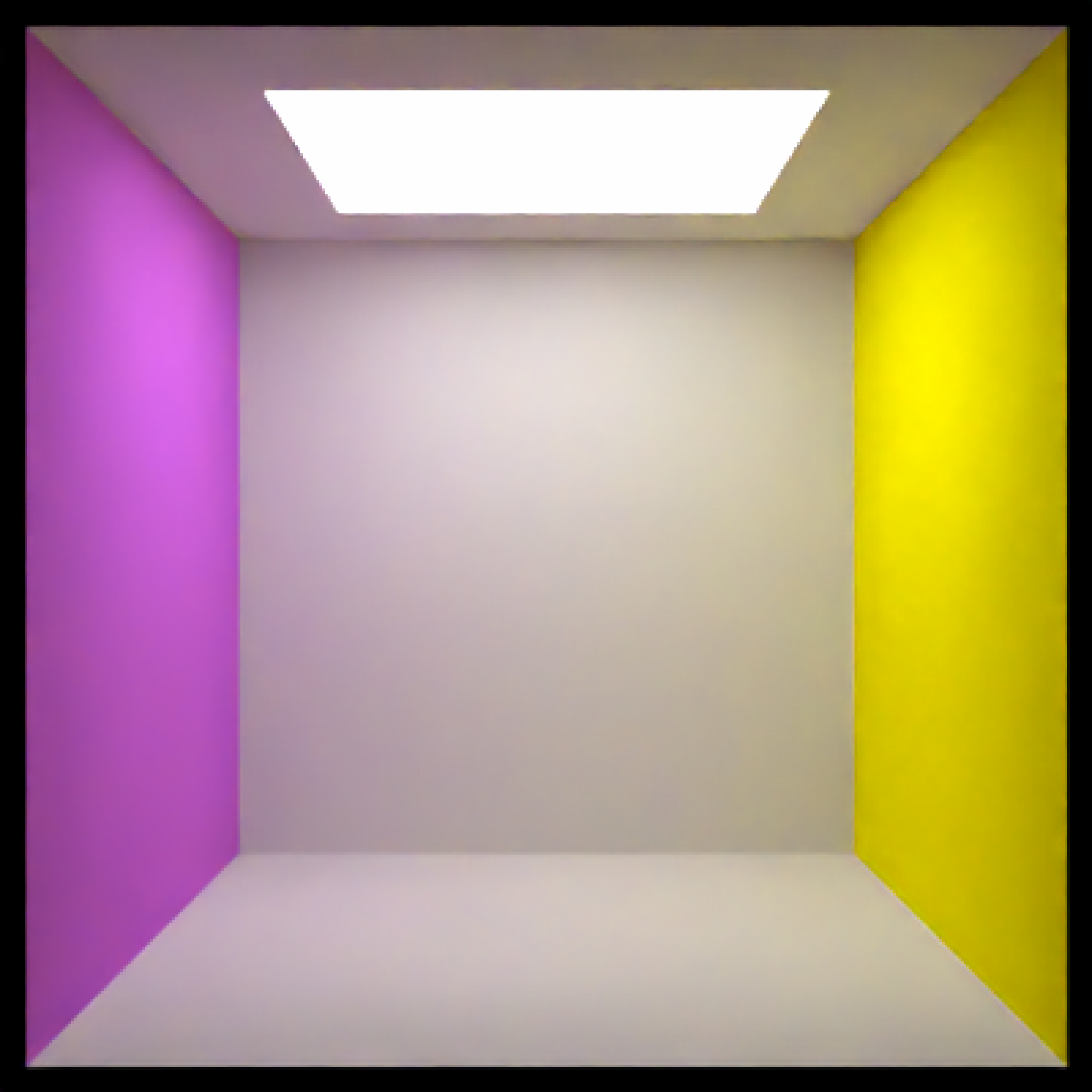Generate Cornell Box

generate_cornell(light = TRUE, lightintensity = 5,
lightcolor = "white", lightwidth = 332, lightdepth = 343,
leftcolor = "#1f7326", rightcolor = "#a60d0d",
roomcolor = "#bababa")

## Arguments

light Default TRUE. Whether to include a light on the ceiling of the box. Default 5. The intensity of the light. Default white. The color the of the light. Default 332. Width (z) of the light. Default 343. Depth (x) of the light. Default #1f7326 (green). Default #a60d0d (red). Default #bababa (light grey).

## Value

Tibble containing the scene description of the Cornell box.

## Examples

#Generate and render the default Cornell box.
scene = generate_cornell()render_scene(scene, samples=200,aperture=0, fov=40, ambient_light=FALSE, parallel=TRUE)#> Setting default values for Cornell box: lookfrom c(278,278,-800) lookat c(278,278,0) .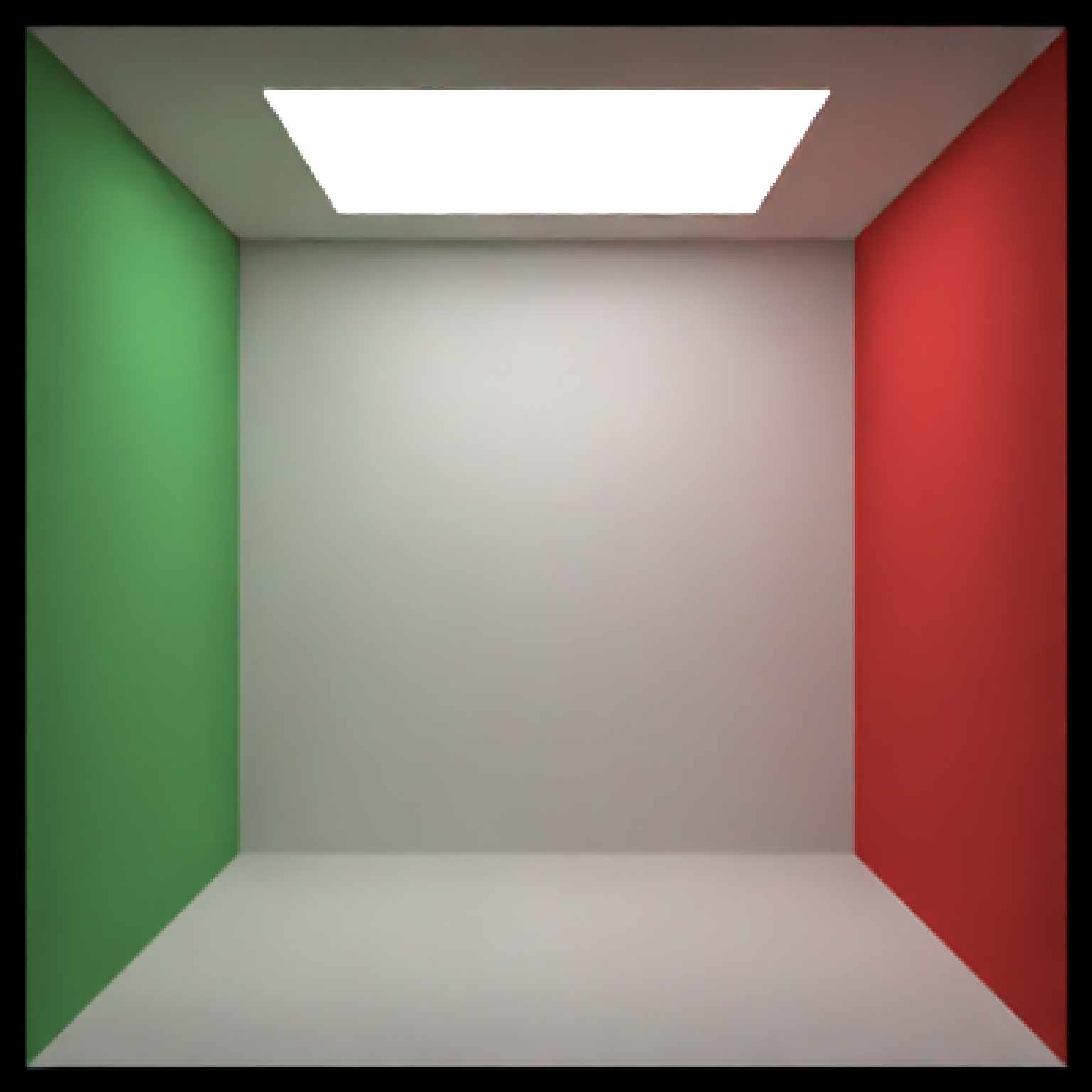#Make a much smaller light in the center of the room.
scene = generate_cornell(lightwidth=200,lightdepth=200)render_scene(scene, samples=200,aperture=0, fov=40, ambient_light=FALSE, parallel=TRUE)#> Setting default values for Cornell box: lookfrom c(278,278,-800) lookat c(278,278,0) .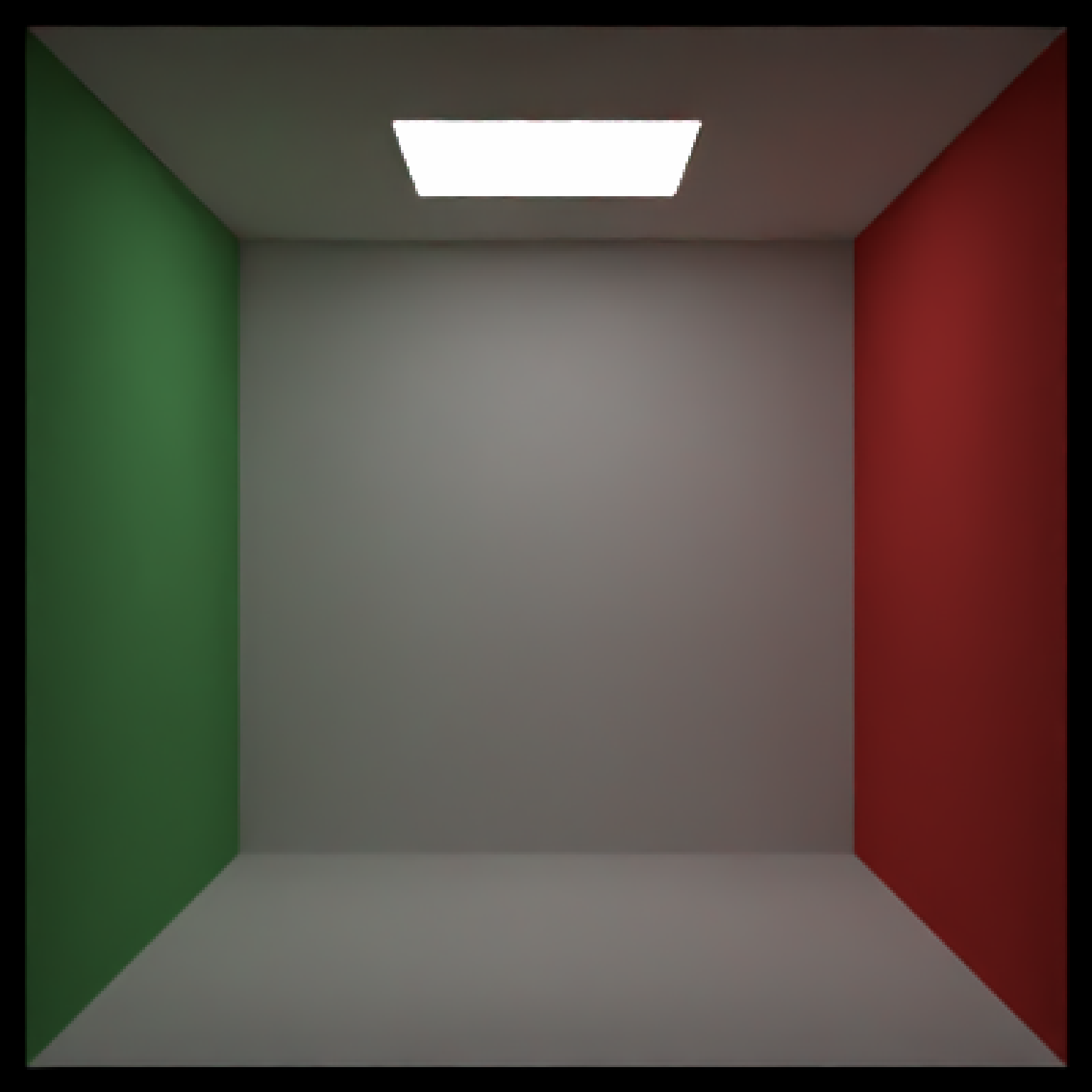#Place a sphere in the middle of the box.
scene = scene %>%
add_object(sphere(x=555/2,y=555/2,z=555/2,radius=555/4))render_scene(scene, samples=200,aperture=0, fov=40, ambient_light=FALSE, parallel=TRUE)#> Setting default values for Cornell box: lookfrom c(278,278,-800) lookat c(278,278,0) .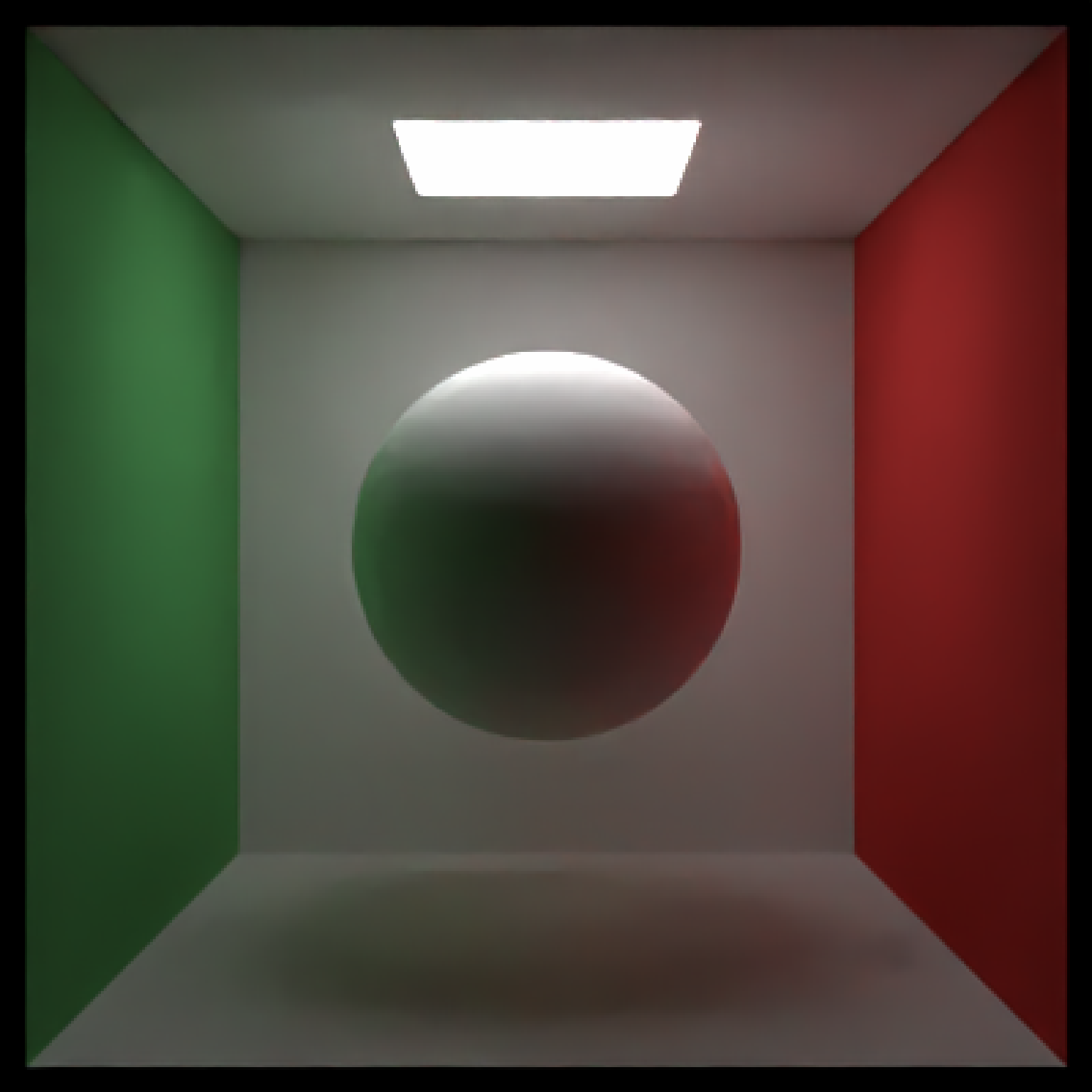#Reduce "fireflies" by setting a clamp_value in render_scene()
render_scene(scene, samples=200,aperture=0, fov=40, ambient_light=FALSE,
parallel=TRUE,clamp_value=3)#> Setting default values for Cornell box: lookfrom c(278,278,-800) lookat c(278,278,0) .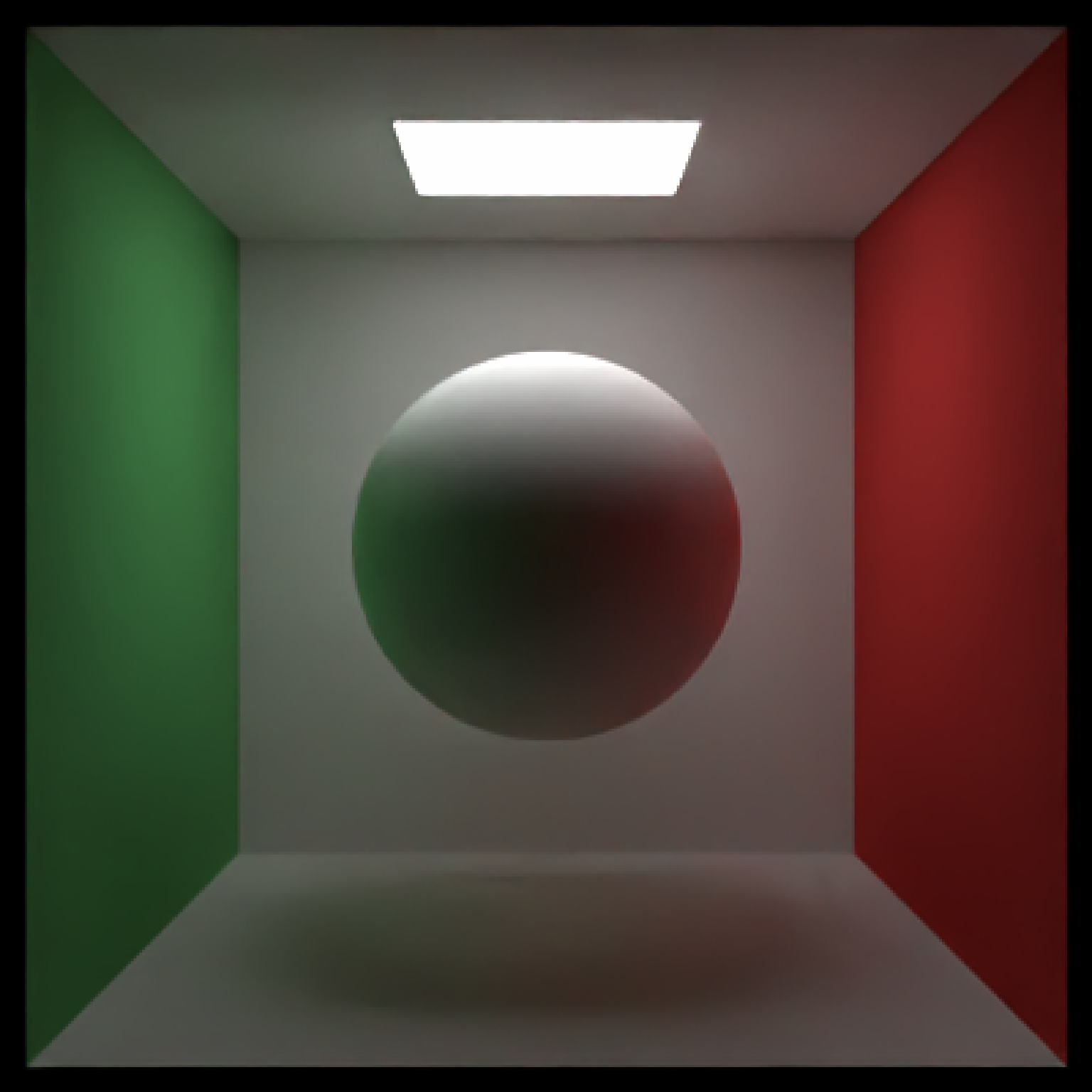# Change the color scheme of the cornell box
new_cornell = generate_cornell(leftcolor="purple", rightcolor="yellow")
render_scene(new_cornell, samples=200,aperture=0, fov=40, ambient_light=FALSE,
parallel=TRUE,clamp_value=3)#> Setting default values for Cornell box: lookfrom c(278,278,-800) lookat c(278,278,0) .